#### Solutions for the average cost-per-stage model

A value iteration algorithm for the average cost model can be obtained by simply neglecting to divide by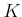. Selecting actions that optimize the total cost also optimizes the average cost as the number of stages approaches infinity. This may cause costs to increase toward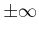; however, only a finite number of iterations can be executed in practice.

The backward value iterations of Section 10.2.1 can be followed with very little modification. Initially, let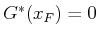for all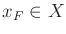. The value iterations are computed using the standard form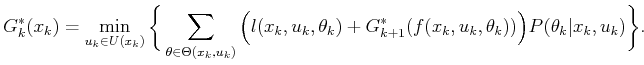(10.78)

The iterations continue until convergence occurs. To determine whether a solution of sufficient quality has been obtained, a reasonable criterion for is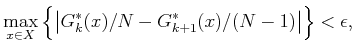(10.79)

in which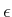is the error tolerance and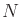is the number of value iterations that have been completed (it is required in (10.80) that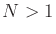). Once (10.80) has been satisfied, the iterations can be terminated.

A numerical problem may exist with the growing values obtained for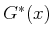. This can be alleviated by periodically reducing all values by some constant factor to ensure that the numbers fit within the allowable floating point range. In , a method called relative value iteration is presented, which selects one state,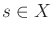, arbitrarily and expresses the cost-to-go values by subtracting off the cost at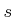. This trims down all values simultaneously to keep them bounded while still maintaining the convergence properties of the algorithm.

Policy iteration can alternatively be performed by using the method given in Figure 10.4 with only minor modification.

Steven M LaValle 2020-08-14